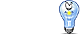Unit Conversion Software Web Widgets Loan Calculator Currency Rates Country Flags Unit Converter
 Faq Help# Watt ConversionContact

## Watt, unit of measure

the SI unit of power. Power is the rate at which work is done, or (equivalently) the rate at which energy is expended. One watt is equal to a power rate of one joule of work per second of time. This unit is used both in mechanics and in electricity, so it links the mechanical and electrical units to one another. In mechanical terms, one watt equals about 0.001 341 02 horsepower (hp) or 0.737 562 foot-pound per second (lbf/s). In electrical terms, one watt is the power produced by a current of one ampere flowing through an electric potential of one volt. The name of the unit honors James Watt (1736-1819), the British engineer who built the first practical steam engines.
To perform conversions between watt and other Power units please try our Power Unit Converter

Convert watt to:
exawatt, petawatt, terawatt, gigawatt, megawatt, kilowatt, hectowatt, dekawatt, deciwatt, centiwatt, milliwatt, microwatt, nanowatt, picowatt, femtowatt, attowatt, horsepower, horsepower (550 ft*lbf/s), horsepower (metric), horsepower (boiler), horsepower (electric), horsepower (water), pferdestarke (ps), Btu (IT)/hour, Btu (IT)/minute, Btu (IT)/second, Btu (th)/hour, Btu (th)/minute, Btu (th)/second, MBtu (IT)/hour, MBH, ton (refrigeration), kilocalorie (IT)/hour, kilocalorie (IT)/minute, kilocalorie (IT)/second, kilocalorie (th)/hour, kilocalorie (th)/minute, kilocalorie (th)/second, calorie (IT)/hour, calorie (IT)/minute, calorie (IT)/second, calorie (th)/hour, calorie (th)/minute, calorie (th)/second, foot pound-force/hour, foot pound-force/minute, foot pound-force/second, pound-foot/hour, pound-foot/minute, pound-foot/second, erg/second, kilovolt ampere, volt ampere, newton meter/second, joule/second, exajoule/second, petajoule/second, terajoule/second, gigajoule/second, megajoule/second, kilojoule/second, hectojoule/second, dekajoule/second, decijoule/second, centijoule/second, millijoule/second, microjoule/second, nanojoule/second, picojoule/second, femtojoule/second, attojoule/second, joule/hour, joule/minute, kilojoule/hour, kilojoule/minuteDownload Power Unit Converter
our powerful software utility that helps you make easy conversion between more than 2,100 various units of measure in more than 70 categories. Discover a universal assistant for all of your unit conversion needs - download the free demo version right away!Copyright © 1998-2009 UnitConversion.org Privacy & Terms | About | Faq | Help | Contact | Link to Us